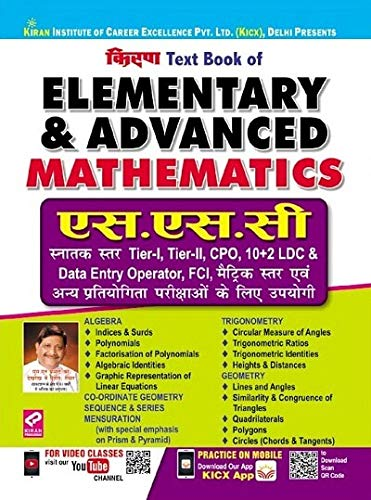# Kiran Elementary And Advanced Mathematics SSC CGL, CPO,CHSL,MTS Exam Hindi (2800)Price: ₹ 328.00
(as of Sep 23,2021 05:45:32 UTC – Details)

Kiran elementary and Advanced Mathematics SSC CGL, CPO, CHSL, MTS exam Hindi (2800) content: Algebra Indices and Surds Polynomials factorization of Quadratic Polynomials Simplification of Fractions algebraic identities solutions of linear Equations graphic representation of straight Lines co-ordinate Geometry sequence and Series important points at a Glance Trigonometry circular measure of angles Trigonometric ratios Trigonometric identities heights and Distances important points at a Glance Geometry lines and angles Triangles: similarity and Congruence Quadrilaterals circles (chords and Tangents important points at a Glance Mensuration area and Perimeter (Triangles, rectangles, squares, quadrilateral, rhombus, circles) surface Area and Volume (Cubes, spheres, hemispheres, cylinders, Cones, frustums, prism, Pyramids).